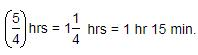Courses

# Test: Boats And Streams- 1

## 10 Questions MCQ Test Quantitative Aptitude (Quant) | Test: Boats And Streams- 1

Description
This mock test of Test: Boats And Streams- 1 for Banking Exams helps you for every Banking Exams entrance exam. This contains 10 Multiple Choice Questions for Banking Exams Test: Boats And Streams- 1 (mcq) to study with solutions a complete question bank. The solved questions answers in this Test: Boats And Streams- 1 quiz give you a good mix of easy questions and tough questions. Banking Exams students definitely take this Test: Boats And Streams- 1 exercise for a better result in the exam. You can find other Test: Boats And Streams- 1 extra questions, long questions & short questions for Banking Exams on EduRev as well by searching above.
QUESTION: 1

### A boat running upstream takes 8 hours 48 minutes to cover a certain distance, while it takes 4 hours to cover the same distance running downstream. What is the ratio between the speed of the boat and speed of the water current respectively?

Solution:

Let the man's rate upstream be x kmph and that downstream be y kmph.

Then, distance covered upstream in 8 hrs 48 min = Distance covered downstream in 4 hrs.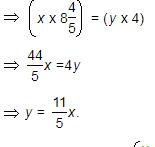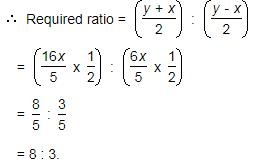QUESTION: 2

### The speed of a boat in still water in 22 km/hr and the rate of current is 4 km/hr. The distance travelled downstream in 24 minutes is:

Solution:

Speed downstream = (22 + 4) kmph = 26 kmph.

Distance travelled = 26 x 24/60

Distance travelled = 10.4 km

QUESTION: 3

### Speed of a boat in standing water is 14 kmph and the speed of the stream is 1.2 kmph. A man rows to a place at a distance of 4864 km and comes back to the starting point. The total time taken by him is:

Solution:

Speed downstream = (14 + 1.2)
= 15.2kmph
Speed upstream = (14-1.2)
= 12.8kmph
Total time taken = 4864/15.2 + 4864/12.8
= 320 + 380
= 700hrs

QUESTION: 4

A motorboat, whose speed in 15 km/hr in still water goes 30 km downstream and comes back in a total of 4 hours 30 minutes. The speed of the stream (in km/hr) is:

Solution:

Let the speed of the stream be x km/hr. Then,

Speed downstream = (15 + x) km/hr,

Speed upstream = (15 - x) km/hr.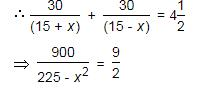⇒  9x2 = 225

⇒  x2 = 25

⇒  x = 5 km/hr.

QUESTION: 5

Q. A man's speed with the current is 15 km/hr and the speed of the current is 2.5 km/hr. The man's speed against the current is:

Solution:

Man's rate in still water = (15 - 2.5) km/hr = 12.5 km/hr.

Man's rate against the current = (12.5 - 2.5) km/hr = 10 km/hr.

QUESTION: 6

A man rows to a place 48 km distant and come back in 14 hours. He finds that he can row 4 km with the stream in the same time as 3 km against the stream. The rate of the stream is:

Solution:

Suppose he move 4 km downstream in x hours. Then,

Speed downstream =Speed upstream =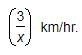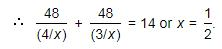So, Speed downstream = 8 km/hr, Speed upstream = 6 km/hr.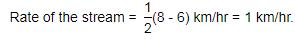QUESTION: 7

A boat can travel with a speed of 22 km/hr in still water. If the speed of the stream is 5 km/hr, find the time taken by the boat to go 54 km downstream.

Solution:

Speed downstream = (22 + 5) km/hr = 27 km/hr.

Time taken to travel 54 km downstream = (54/27) hours

= 2 hours

QUESTION: 8

In one hour, a boat goes 14 km/hr along the stream and 8 km/hr against the stream. The speed of the boat in still water (in km/hr) is:

Solution:

Speed in still water = 1/2 (14 + 8) km/hr

= 11 km/hr

QUESTION: 9

In one hour, a boat goes 14 km/hr along the stream and 8 km/hr against the stream. The speed of the boat in still water (in km/hr) is:

Solution:

Speed in still water =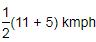= 8 kmph.

QUESTION: 10

A boatman goes 2 km against the current of the stream in 1 hour and goes 1 km along the current in 10 minutes. How long will it take to go 5 km in stationary water?

Solution:

Rate downstream =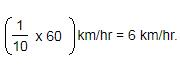Rate upstream = 2 km/hr.

Speed in still water =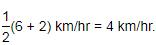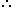Required time =